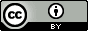Sunday, August 16, 2015

Finding the melting temperature by coexistence simulations

While reviewing a paper I came across the idea of finding melting temperatures by coexistence simulations.  The idea is very simple:

1. Run an $NVE$ MD simulation starting from a configuration where half the molecules are solid and the other half is liquid.
2. If the choice of $E$ is such that both phases exist after equilibrium then the average temperature will of the system will converge to the melting temperature.
3. In practice you determine $E$ by running a short $NVT$ MD simulation, where $T$ is reasonably close to the suspected melting temperature, and using the final position and velocities as initial conditions for the $NVE$ simulation.  It's probably best to use a range of temperatures.
4. If you want the melting temperature at constant $P$ run an $NPH$ MD simulation instead.

I traced the approach back as far as this paper, which also has a nice explanation of why this works:
A more direct approach (Ref) to finding the transition temperature is to avoid the nucleation problem altogether, i.e., by simulating coexisting phases and allowing the system to evolve to equilibrium. If the equilibrium system contains both solid and liquid phases, then the system will be at a melting point. This approach is suitable for both experimental and theoretical studies. Molecular dynamic (MD) techniques are particularly useful for this approach, due to the fact that total energy is conserved in conventional MD schemes. To understand how this helps the system evolve toward equilibrium, consider a system with a phase boundary. If the system as a whole is at a temperature slightly below the melting point, then some portion of the liquid phase will solidify, generating the appropriate latent heat. Because the system is closed, this heats up the system towards the melting point. Similarly, if the system is above the melting temperature, the latent heat required to melt the solid will cool the system. The pressure of the system will also tend to equilibrate; thus, the system will evolve toward an equilibrium phase. There is no difficulty in nucleating either the liquid or solid phases, as the interface assists in the nucleation for the melting or solidification process.
The paper references an earlier book chapter, which I didn't bother to get a hold of,* that might reference even earlier works.  (*yet another demonstration of why publishing original work as a book chapter is equivalent to burying it in your backyard).